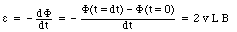## Solutions Physics 122 Midterm Exam # 3

Problem 1

The magnetic moment of the coil is equal to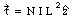where N is the number of turns, I is the current, and L is the length and width of the coil. The torque exerted on the coil is equal to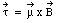The maximum torque is obtained when the magnetic field and the magnetic moment are perpendicular to each other. This maximum value is equal to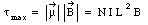Problem 2

Using the right-hand rule and the symmetry of the U-shaped wire, we can conclude that the forces on the long sides of the U-shaped wire cancel. The total force acting on the U-shaped wire is thus equal to the force acting on the section that is parallel to the long straight wire. The magnitude of the magnetic field generated by the long straight wire at this position is equal to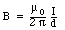The magnetic field is directed out of the paper, perpendicular to the short section of the U-shaped wire. The force acting on the U-shaped wire is equal to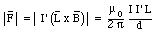Problem 3

The rod moves to the right. As a consequence, the magnitude of the enclosed magnetic flux is reduced. Lenz' law indicates that the induced current will generate a magnetic field that opposes this change. The induced current will thus produce a magnetic field that is directed into the paper. The induced current will therefor need to flow clockwise. Inside the rod the current will flow from B to A. The magnetic force acting on the rod will therefor be directed towards the left and if the rod is moving with a constant velocity, a constant force directed towards the right must be supplied. Thus:

• Using the right-hand rule we can conclude that the induced current must flow clockwise.
• The magnitude of the magnetic flux through ABCD will decrease.
• If we consider a positive charge in the rod, then the motion towards the right will produce a magnetic force on the charge directed towards A. As a consequence, there will be an induced positive charge at A compared to B.
• A constant force is required to keep the rod moving to the right with a constant velocity.
• The magnetic field due to the induced current points into the plane of the paper.

Problem 4

Consider the situation shown in the Figure on the assignment. The direction of the current is perpendicular to the direction of the magnetic field, and the resulting magnetic force is directed vertically downwards. The wire will thus move down until the magnetic force is balanced by the spring forces. In the equilibrium position the springs will be stretched.

If the current is reversed, the magnetic force acting on the wire will be directed vertically upwards. The wire will thus move up until the magnetic force is balanced by the spring forces. In the new equilibrium position the springs will be compressed.

If the direction of the magnetic field is changed such that it is now pointing vertically downwards, then the magnetic force acting on the wire will be directed horizontally into the paper. The wire would thus move into the page to a new equilibrium position.

If the direction of the magnetic field is parallel to the wire then there is no magnetic force acting on the wire. As a result, the wire will not move when the current is turned on. However, if we start with the current being on, the change in direction of the magnetic field will move the wire from its "downwards" equilibrium position to its "rest" position, which is the position where the wire is located when no current is flowing through the circuit.

If the direction of the magnetic field is reversed to that it is pointing horizontally into the page, the direction of the magnetic force acting on the wire will also reverse, and will not be directed vertically upwards. As a result, the wire will move up to a new equilibrium position.

Problem 5

The magnetic field inside the long solenoid is equal to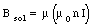where u is the permeability of the ferromagnetic material. The current I required to produce a magnetic field B is thus equal to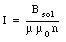Problem 6

In the steady state (dI/dt = 0 A/s), the potential drop across the inductor is equal to 0 V, and the current is constant and independent of time, with a value of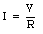The energy stored in the inductor is equal to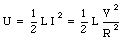Problem 7

To solve this problem we need to use a phasor diagram (see Figure).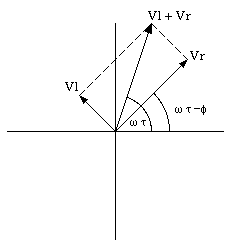Figure Problem 7.

The voltage across the resistor is equal to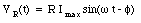The voltage across the inductor is equal to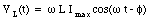The length of the phasor representing the voltage across the resistor is equal to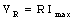The length of the phasor representing the voltage across the inductor is equal to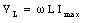Based on the phasor diagram shown in the Figure we can conclude that the phase angle [phi] is equal to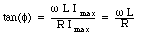Thus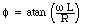Problem 8

Consider a clockwise induced emf. If this emf is defined as a positive emf then the flux associated with a magnetic field pointing in the positive z-direction will be positive, while the flux associated with a magnetic field pointing in the negative z-direction will be negative. When the center of the loop is located at the origin, the total enclosed magnetic flux is equal to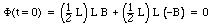After a time dt, the center of the loop will have moved to x = v dt. At this time the enclosed flux is equal to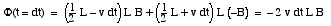The induced emf is thus equal to